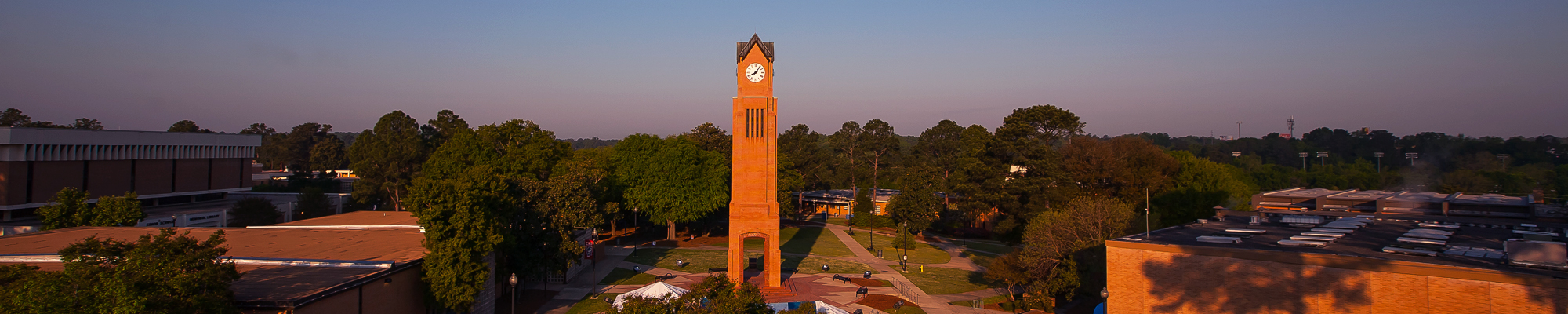## Compare Courses

STAT 1127. Introductory Statistics (3-0-3) Prerequisite: Satisfactory score on math placement exam or completion of any of the following courses with a grade of "C" or better: MATH 1001, MATH 1101, MATH 1111, MATH 1113, MATH 1125, or MATH 1131. Survey of modern statistical methods applicable to behavioral, biological, health and managerial sciences, and education. Organization and analysis of data, probability distributions, sampling distributions, point estimation, confidence interval, hypothesis testing, and regression analysis. (Course fee required.)

STAT 3127. Statistical Computing (3-0-3) Prerequisite: STAT 1127 with a grade of "C" or better. The goal of this course is to provide students with an introduction to SPSS programming for data management, analysis, and reporting, and familiarize students with practical issues related to the exploration of actual data sets. This course introduces the most commonly used features of SPSS, especially in examining, transforming, and analyzing data (linear regression, ANOVA, and dummy variable regression).

STAT 5117. Applied Multivariate Analysis (3-0-3) Prerequisite: STAT 3127 with a grade of C or better. Applied multivariate methods, sample correlations, multivariate date plots, eigenvalues and eigenvectors, principle components analysis, factor analysis, discriminant analysis, logistic regression methods, cluster analysis, mean vectors and variance-covariance matrices, multivariate analysis of variance, prediction models.

STAT 5118. Applied Nonparametric Methods (3-0-3) Prerequisite: STAT 3127 with a grade of "C" or better. Rank tests of comparing two treatments, comparing two treatments or attributes in a population model, blocked comparisons for two treatments, paired comparisons in a population model and the one-sample problem, the comparison of more than two treatments, randomized complete blocks, tests of randomness and independence.

STAT 5119. Applied Categorical Data Analysis (3-0-3) Prerequisite: STAT 3127 with a grade of "C" or better. Sampling distributions, two by two contingence tables, Simpson's paradox and 2ˆ3 Tables, Goodman's full rank interaction analyzed for two way tables, further examples and extensions, conditional independence models for two-way tables, further topics.

STAT 5176. Statistical Design and Analysis of Experiments (3-0-3) Prerequisite: STAT 3127 with a grade of "C" or better. Completely randomized designs, treatment comparisons, diagnosing agreement between the data and the model, experiments to study variances, factorial treatment design and applications. Appropriate statistical software will be used.

STAT 5177. Applied Regression Analysis (3-0-3) Prerequisite: STAT 3127 with a grade of "C" or better. Simple and multiple regression, transformation of variables, diagnostic procedures, analysis of variance and residuals, comparison of two multiple regression models, calibration and regulation for linear regression, linear splines, subset analysis and variable selection, nonlinear regression. Appropriate statistical software will be used.

STAT 5555. Selected Topics in Statistics (3-0-3) Prerequisite: STAT 3127 with a grade of "C" or better. Logistic and Probit analyses in problems of assay. Count data analysis. Methods of survival analysis. Analysis of contingency tables, Analysis of variance for balanced data, unbalanced data, repeated measures data, binomial data.